Next: Examples of MIS Learning Up: COMP9417 Project Shapiro's Model Previous: The Contradiction Backtracing Algorithm

Subsections

# Refinement

We saw in Section 2 how MIS goes about specialising a theory that is too general; the counterpart problem of generalising a theory that is too specific is carried out with the use of a refinement operator.

The following inputs are required:

• A theory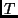that fails to prove a positive observation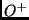. We say thatis too-specific; it is incomplete relative to the concept the oracle has in mind.

• An oracle capable of answering queries about the truth of ground atoms in the model.

• A set of marked clauses that the backtracing algorithm has previously deemed too general to describe.

• A refinement operator, that, when applied to a marked clause, yields a logically weaker (i.e. more specific) clause.

It should be noted that, even though the new candidate clause is less general than the previously-considered one, adding it does not decrease the number of facts entailed by the current theory. (It may not actually increase it, though.) So, the new conjecture should be considered a generalisation of the previous one.

Shapiro's generalisation algorithm is parameterised by both the refinement operator and the strategy that makes use of it. We deal with each in the following sections.

## Refinement Operators

A refinement operatorsuggests a logically weaker plausible replacement to a hypothesis refuted by the diagnosis algorithm outlined in Section 2. Formally,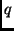is a refinement of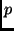if it satisfies the following two criteria:

1.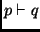, i.e. everything provable fromis provable from, or equivalently,is at least as general as.

2.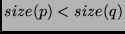under some size metric. In logic programming terms, Shapiro uses the following:
The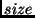of a sentence,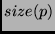, is the number of symbol occurrences in(excluding punctuation symbols) minus the number of distinct variables occurring in.

Intuitively, building a monotonic size increase into the notion of refinement means that all refinements ofare more specific/weaker than, as it requires more things to be provable (in the case of adding a body goal) or more structure to be present in the query (if a function symbol is introduced, or two or more variables are unified). This exploits the intimate relationship between the syntax and semantics of logic programs.

A refinement operatoris then defined to be a set-valued function that maps a statementto a subset of its refinements, with some technical side-conditions.

A function satisfying this definition induces a partial order (where larger'' is interpreted to mean more-specific) over the statements of the language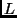, and can be visualised as a directed acyclic graph (generally termed a Hasse diagram in the context of partial orders). If all statements inappear in the graph at some point, and are transitively reachable from the most-general clause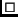, then the refinement operator is termed complete for the language.

Shapiro proves the existence of a refinement operator complete for any first order language in [Sha81]. This property in itself is not particularly desirable, however, as it leads to inefficient induction of theories due to the exponential explosion of the size of the refinement graph. It is more interesting to define refinement operators that work on subsets ofthat are more likely to contain satisfactory theories, as this is the only way the search is going to be tractable.

One class of interesting logic programs that can be enumerated via a refinement operator in practice is the that of the definite clause grammars. Using such an operator, it is possible to learn the syntax of simple programming languages.

The refinement operator used in Section 4 is defined as follows: Let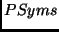be the set of predicate symbols in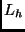,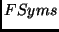the function symbols; then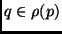iffis a modification ofin precisely one of the following ways:

1. Let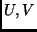be distinct variables in; unify them in(i.e.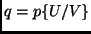, read as substitute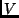for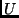in'').

2. Let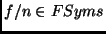andbe a variable in; let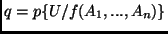where theare new variables.

3. Let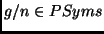,distinct variables inand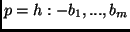; let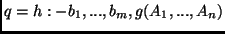.

See Section 4.1 for a discussion of some of the pragmatic issues of defining a useful refinement operator.

Figures 3 and 4 show how the two clauses for the add/3 relation can be derived using these rules. Note that in practice there will probably be several other clauses included in the theory, such as the symmetric base case add(B, z, B).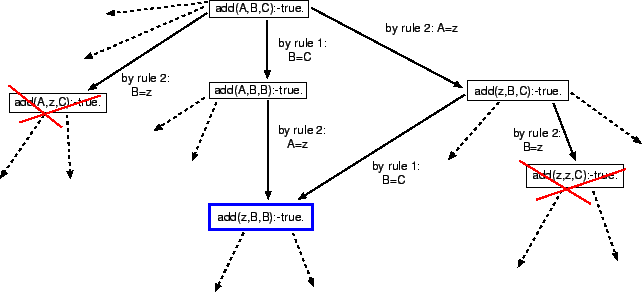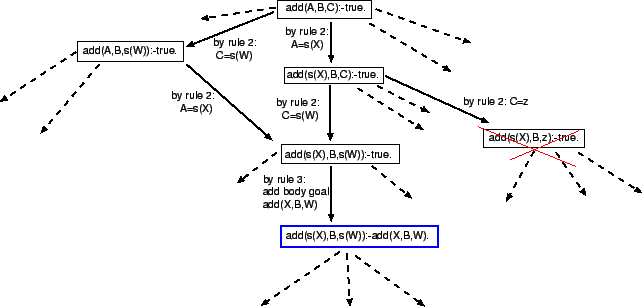## Using Refinement Operators

In essence, the bug-correction algorithm employed by MIS is little more than a search through an enumeration of all clauses in. Given the intractability of this search in general, the given refinement operatoris used to bias the enumeration towards clauses that are more likely to be correct.

The search itself is general-to-specific, starting with the empty theory. The advantage of this strategy over a specific-to-general one is that there is a simple starting point; for the latter the initial theory would have to contain - notionally, at least - all ground statements in. This set will be infinite if there is at least one function symbol with an arity of one or more in the language (which will be the case in all interesting domains), and so is difficult to represent on a physical machine.

Asinduces a partial (and not total) order on, three sets need to be maintained:

• The theory set contains all clauses that have been examined and found to be consistent with the facts.

• The marked set contains all clauses that have been flagged by the contradiction backtracing algorithm as the culprits for the proof of a negative observation, and have yet to be refined.

• The dead set contains all marked clauses that have been refined.

The search itself is straightfoward: select the next marked clause, add it's refinements to the theory and move it to the dead set. Note that the ordering of the marked set must be fair, for otherwise the algorithm may get stuck examining an infinite path that does not contain useful clauses. This is analogous to a Prolog proof attempt failing to terminate even though the query logically follows from the program, due to it's incomplete depth-first-search strategy.

In the context of Figure 1, searching the refinement DAG is purely a generalisation step; the consistency of the resulting theory with the facts is established by the framework itself.Next: Examples of MIS Learning Up: COMP9417 Project Shapiro's Model Previous: The Contradiction Backtracing Algorithm
Peter Gammie
2002-03-01